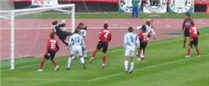# Rounding Numbers

## What is "Rounding" ?

Rounding means making a number simpler but keeping its value close to what it was.

The result is less accurate, but easier to use.Example: 73 rounded to the nearest ten is 70, because 73 is closer to 70 than to 80. But 76 goes up to 80.

## Common Method

There are several different methods for rounding. Here we look at the common method, the one used by most people.

First some examples (explanations follow):

## How to Round Numbers

• Decide which is the last digit to keep
• Leave it the same if the next digit is less than 5 (this is called rounding down)
• But increase it by 1 if the next digit is 5 or more (this is called rounding up)

### Example: Round 74 to the nearest 10

• We want to keep the "7" (it is in the 10s position)
• The next digit is "4" which is less than 5, so no change is needed to "7"

(74 gets "rounded down")

### Example: Round 86 to the nearest 10

• We want to keep the "8"
• The next digit is "6" which is 5 or more, so increase the "8" by 1 to "9"

(86 gets "rounded up")

So: when the first digit removed is 5 or more, increase the last digit remaining by 1.

## Why does 5 go up ?

5 is in the middle ... so we could go up or down. But we need a method that everyone agrees to.

So think about sport: we should have the same number of players on each team, right?0,1,2,3 and 4 are on team "down" 5,6,7,8 and 9 are on team "up"

And that is the "common" method of rounding. Read about other methods of rounding.

A farmer counted 87 cows in the field, but when he rounded them up he had 90.

## Rounding Decimals

First work out which number will be left when we finish.

• Rounding to tenths means to leave one number after the decimal point.
• Rounding to hundredths means to leave two numbers after the decimal point.
• etc.

### 3.1416 rounded to hundredths is 3.14

as the next digit (1) is less than 5

### 3.1416 rounded to thousandths is 3.142

as the next digit (6) is more than 5

### 1.2735 rounded to tenths is 1.3

as the next digit (7) is 5 or more

To round to "so many decimal places" count that many digits from the decimal point:

### 1.2735 rounded to 3 decimal places is 1.274

as the next digit (5) is 5 or more

## Rounding Whole Numbers

We may want to round to tens, hundreds, etc, In this case we replace the removed digits with zero.

### 134.9 rounded to tens is 130

as the next digit (4) is less than 5

### 12,690 rounded to thousands is 13,000

as the next digit (6) is 5 or more

### 15.239 rounded to ones is 15

as the next digit (2) is less than 5

## Rounding to Significant Digits

To round to "so many" significant digits, count digits from left to right, and then round off from there.

### 1.239 rounded to 3 significant digits is 1.24

as the next digit (9) is 5 or more

### 134.9 rounded to 1 significant digit is 100

as the next digit (3) is less than 5

When there are leading zeros (such as 0.006), don't count them because they are only there to show how small the number is:

### 0.0165 rounded to 2 significant digits is 0.017

as the next digit (5) is 5 or more## Significant Digit Calculator

(Try increasing or decreasing the number of significant digits. Also try numbers with lots of zeros in front of them like 0.00314, 0.0000314 etc)